# 1st PUC Statistics Question Bank Chapter 10 Random Variables and Mathematical Expectation

## Karnataka 1st PUC Statistics Question Bank Chapter 10 Random Variables and Mathematical Expectation

### 1st PUC Random Variables and Mathematical Expectation Two Marks Questions and Answers

Question 1.
Define Random variable.
Random variable is a function which assigns a real number to every sample point in the sample space.

Question 2.
Define discrete random variable
A random variable ‘ X ’ which takes the specified values x1x2,…,xn with a respective probabilities p1, p2,….., p1 is a discrete random variable.

Question 3.
Define continuous random variable.
A random variable which assumes all the possible values in its range is called a continuous random variable.

Question 4.
Define Mathematical expectation of a random variable/Mean of random variable.
Let ‘X’ be a discrete random variable which can takes the values x1, x2, x3,… ,xn with respective probabilities p1, p2,p3,… , pn then, the mathematical expectation of ‘ X ’ is:E(X) = x1p1 + x2P2 + x3P3 + – + xnPn
Then E(X) = Σ x p(x) ;it is also called as mean of discrete random variable (X)Question 5.
Define probability distribution.
A systematic presentation of the values taken by a random variable with respective probabilities is called the probability distribution of a random variable’.

Question 6.
Probability mass function (pmf).
Probability mass function (pmf): Let ‘ X ’ be a discrete random variable . And let p(x) be a function : p(x) = p(X=x) . Then p(x) is called the probability mass function of ‘ X ’. If the following conditions are satisfied,

• p(x) ≥ 0 for all values X, and
• Σ p(x) =1.

Question 7.
Probability density function (pdf).
Probability density function (pdf): Let ‘ X ’ be a continuous random variable taking values in the interval [a, b], then,
A function f(x) is said to be the probability density function of the continuous random variable ‘ X ’, if it satisfies the following conditions :

• f(x) ≥ 0 for all ‘ X’ in the interval [a, b] .
• For two distinct numbers c & d in the interval [a, b]:
P(c ≤ X ≤ d) = (Area under the probability curve between ordinates at X =c and X=d).
• Total area under the curve is 1. i.e. , P(- ∞ < x < ∞) = 1 .

Question 8.
Writedown the formulae of all Mathematical Expectations:

• E(X) = XΣX.P (X)
• E(a) = a,
• E(aX) = a E(X) and
• E(aX+b) = aE(X)+b.

Question 9.
Write down the Formulae of Variances:

• Var(X)= {E(X2)-[E(X)]2} ,
• V (a)=0,
• V(aX)=a2 V(X),
• V(aX+b)=a2V(X), and S.D.(X)= $$\sqrt{\mathrm{v}(\mathrm{x})}$$.

Question 10.
Write down the Formula of Co-Variance:
Cov(X, Y) =E(XY) – E(X).E(Y),

Question 11.
Write down the co-efficient of correlation: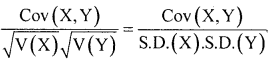Question 12.
Statement: Let X and Y be two random variables with respective expectations E(X) and E(Y). Then, E(X + Y)=E(X) + E(Y)

Question 13.
State Multiplication of expectation.
statement: Let X and Y be two independent random variables with respective expectations E(X) and E(Y). Then expectation of the product of these random variables is; E(XY) = E(X) E(Y)

Question 14.
If E(X)=2, then find the value of E(-x/4)
Given E(-x/4) = E(-l/4. x) =-l/4.E(X) = -1/4. 2 =-1/2 since E(aX) = a E(X)

Question 15.
If E(X)=2/5, find E(5x/3)
Given E(5x/3) = E(5/3.x) = 5/3.E(x) = 5/3.2/5 = 2/3Question 16.
If X is a random variable and E(X) =1.5, then what is the value of E(3 + 4X)?
E(3 + 4X) = E(4x + 3) = 4.E(x) + 3 = 4.(1.5) + 3 = 6 +3 = 9 since
E(ax+b) = aE(x)+b

Question 17.
If E(X) = 2, find the value of E(3X – 6).
E(3X – 6) = 3E(x) – 6 = 3(2) – 6 =6 – 6 =0

Question 18.
If E(X+Y) =7 and E(X) =4, then find the value of E(Y).
We know that E(X+Y) = E(X) + E(Y) ;
7 = 4 + E(y)
E(y) = 7 – 4 = 3

Question 19.
If E(X) = 3 and E(Y) = 5, then find E(3X+2Y), E(3X -Y)
E(3X + 2Y) = 3 E(x) + 2 E(y) = 3(3) + 2(5) = 9 + 10=19
E(3X – Y) = 3 E(X) + (-1) E(Y) = 3(3)- 5 = 9 – 5 = 4

Question 20.
If X and Y are two independent random variable, E(XY)=10 and E(X)= 4, then what is the value of E(Y)?
We know for any two independent random variables:
E(XY) = E(X)E(Y);
10 = 4 E(Y); E(Y)= 10/4 = 2.5

Question 21.
What is the value of co-efficient of correlation for two independent variables?
Zero ie., r = 0

Question 22.
For a random variable X, E(X2) = 50 and V(X)=14, then what is E(X)?
We know that V(X) = E(X2)-[E(x)]2
14 = 50-[E(x)]2
[E(x)]2 = 50 – 14 = 36
Squaring on both sides, we get E(x) = $$\sqrt{36}$$ = 6

Question 23.
If E(X)=3 and E(X2)=45, find the value of S.D.
We know S.D(x)
$$\begin{array}{l}{=\sqrt{\mathrm{V}(\mathrm{x})}=\sqrt{\mathrm{E}(\mathrm{X} 2)-[\mathrm{E}(\mathrm{x})] 2}} \\ {=\sqrt{45-^{2}=\sqrt{45-9}}=\sqrt{36}=6}\end{array}$$

Question 24.
If E(X) = 4, E(X2) = 25, find V(5X – 9).
V(5x-9)this is of the type V(aX+b)=a2V(X)
V(5x-9) = 52. V(x)
Here V(X) = E(X2) – [E(x)]2;
= 25 – 42 = 25 – 16 = 9
∴ V(5×-9) = 52. V(x) = 25(9) = 225

Question 25.
If V(X)=4 then find V(2X)
V(2x) = 22V(x) = 4 (4) = 16; Since V(aX) = a2 V(X)Question 26.
If V(X) = 5 then find V(-5X).
V(-5X) = (5)2 V(x) = 25(5) = 125; Since V(aX)=a2V(X)

Question 27.
For a random variable V(X) = 4. Find V(3X +4) and V(-3X).
V(3X+4) = 32 V(x) = 9 (4) = 36;
V(-3X) = (-3)2 V(x) = 9 (4) = 36; Since V(aX+b)=a2 V(X),

Question 28.
If V(x)=1.6, then find V(2X – 5).
V(2X-5) = 22 V(x) = 4 (1.6) = 6.4; Since V(aX+b)=a2 V(X),

Question 29.
If E(X) = 5, E(Y) = 2, E(XY) = 11, then find Cov(X,Y).
We know that Cov(X, Y) = E(XY) – E(X).E(Y)
= 11 – (5) (2)=11 -10= 1.

Question 30.
When X and Y are two discrete random variables with E(X)=5, E(Y)=6, E(X2)=34, E(Y2)=52, and E(XY)=25, then find Cov(X,Y) andin a bi-variate data
Cov (X, Y) = E(XY)-E(X).E(Y) = 25 – (5) (6) = 25 – 30 = -5;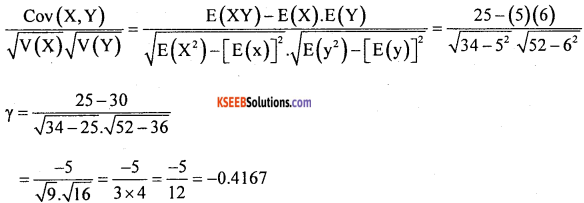Question 31.
If V(X)=V(Y)=Cov(X,Y), then what is the value of ?
γ = 1

Question 32.
If P(X = 3) = 2/3 and P(X = -2) = 1/3, then find E(X) and V(3X + 4)
Given probability distribution is: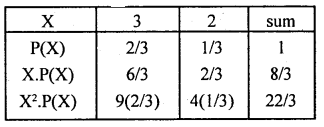E(x) =8/3;
V(x) = E(X2)-[E(X)]2
= – (8/3)2
= 22/3-7.0756 = 7.3333-7.0756 = 0.2577
V(3X + 4) = 32. V(X) = 9 (0.2577) = 2.3193

Question 33.
If P(X) is a probability mass function (p.m.f) then what is ΣP (x)?
ΣP(X)=1

Question 34.
Find the mean and standard deviation for the following probability distribution.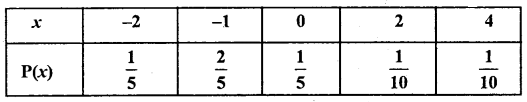We Know that : Mean = E(x) : Σx.P(X)=1
$$=(-2) \times \frac{1}{5}+(-1) \times \frac{2}{5}+0 \times \frac{1}{5}+2 \times \frac{1}{10}+4 \times \frac{1}{10}$$
E(x) =-0.4 -0.4 + 0 +0.2+ 0.4 = -0.2
Var(x) = E(x2) – [E(x)]2
Here E(x2) = [x2. P(x)]
$$=(-2)^{2} \times \frac{1}{5}+(-1)^{2} \times \frac{2}{5}+0^{2} \times \frac{1}{5}+2^{2} \times \frac{1}{10}+4^{2} \times \frac{1}{10}$$
= 0.8+ 0.4+ 0 + 0.4+ 1.5
E(x2) = 3.2
∴ Var(x) =3.2-(-0.2)2 = 3.2 – 0.04 = 3.16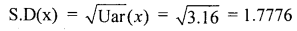Question 35.
If two coins are tossed once. Find the mean and variance of number of Heads.
S = {HH, HT, TH, TT}
The probability of number of Heads is :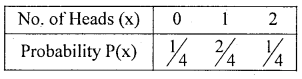E(x) = Σx. P(x) = $$0 \times \frac{1}{4}+1 \times \frac{2}{4}+2 \times \frac{1}{4}$$
Var (x) = E(X2) [E(x)]2
E(x2) = Ex2. P(x) .
$$=0^{2} \times \frac{1}{4}+1^{2} \times \frac{2}{4}+2^{2} \times \frac{1}{4}=0+0.5+1=1.5$$
∴ Var (x) = 1.5 – 12 = 0.5

Question 36.
A fair die thrown once. A person will gets ₹ 5 if the faces of a die results in mulitple of 2. other wise he loses ₹ 2. find his expected amount.
Let x denote the expected amount is random variable which can take: 5 (die results in multiple of 2 : 2.46) and -2( die results in 1, 3, 5), with respective probabilities.
P(x = 5) = P(getting multiple of 2) = $$\frac{3}{6}=\frac{1}{2}$$
P(x = -2) = P(Not getting multiple 2) = $$\frac{3}{6}=\frac{1}{2}$$
The probability distribution is :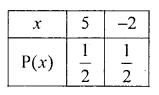Expected amount: E(x) = Σx. P(x) = $$5 \times \frac{1}{2}-2 \times \frac{1}{2}=2.5-1$$

Question 37.
A person tosses a fair coin, he gets ₹ 50 if head turns up and loses ₹ 10 if tail appears. Find the expected amount, also find the standard deviation.
Let x denote the person to gets the amount is a random variable, which takes the values 50 and -10, with respective probabilities:
P(x = 50) = P(getting Head) = $$\frac { 1 }{ 2 }$$ and P(x = -10) = P(getting tail) = $$\frac { 1 }{ 2 }$$
The probability distribution is :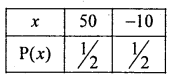The expected amount: E(x) = Σx. P(x) = 50 × $$\frac { 1 }{ 2 }$$ -10 × $$\frac { 1 }{ 2 }$$ = 25 – 5 = ₹20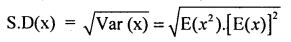Here E(x2) = Ex2. P(x) = (50)2 × $$\frac { 1 }{ 2 }$$ + (-10)2 × $$\frac { 1 }{ 2 }$$
E(x2) = 1250 + 50= 1300
∴ S.D(x) = $$\sqrt{1300-(20)^{2}}=\sqrt{900}$$ =₹ 30.

Question 38.
A box has 5 blue and 3 yellow marbles. Two marbles are drawn at random. Find the expected number of blue marbles drawn.
Let x denote the number of blue marbles drawn, is a random varible which takes the values: 0, 1,2. The respective probabilities are calcualted as below:
P(x = 0) = P(drawing no blue marbles)
= P(2 yellow marbles) = $$\frac{^{3} \mathrm{C}_{2}}{^{8} \mathrm{C}_{2}}=\frac{3}{28}$$
P(x = 1) = P(drawing one blue and one yellow marbles)
$$=\frac{^{5} \mathrm{C}_{1} \times^{3} \mathrm{C}_{1}}{^{8} \mathrm{C}_{2}}=\frac{15}{28}$$
P(x = 2) = P(drawing two blue marbles) = $$\frac{^{5} \mathrm{C}_{2}}{^{8} \mathrm{C}_{2}}=\frac{10}{28}$$
Then the probability distribution is :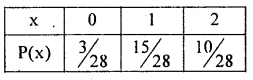Expected number of blue marbles: E(x) = Σx P(x)
$$=0 \times \frac{3}{28}+1 \times \frac{15}{28}+20 \times \frac{10}{28}=0+0.5357+0.3571$$
∴ E(x) =0.8928

Question 39.
The following is the probability distribution of x. Find k and E(x).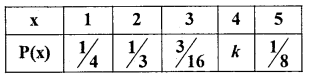For a probability mass funciton : EP(x) = 1
$$\text { i.e., } \frac{1}{4}+\frac{1}{2}+\frac{3}{10}+k+\frac{1}{8}=1$$
$$\mathrm{k}=1-\left[\frac{1}{4}+\frac{1}{2}+\frac{3}{10}+\mathrm{k}+\frac{1}{8}\right]$$ ∴K = 1 – 0.8925 = 0.1075
∴ The probability distribution is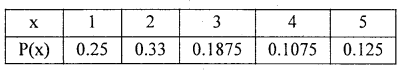E(x) = Σx. P(x) = (1 × 0.25) + (2 × 0.33) + (3 × 0.1875) + (4 × 0.1075) + (5 × 0.125) = 2.5275

Question 40.
From the following probability distribution of x, Find k, E(x), E(x + 3) and Uar (x).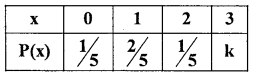By the definition of p.m.f: Σ P(x) = 1
$$\therefore \quad \frac{1}{5}+\frac{2}{5}+\frac{1}{5}+k=1$$  $$\left.\mathrm{k}=-1-\left[\frac{1}{5}+\frac{2}{5}+\frac{1}{5}\right]=0.2+0.4+0.2\right]$$
k = 1 – 0.8 = 0.2
To find E(x), E(2x + 3), (x) and (2x + 3) we need compute from the probability distribution.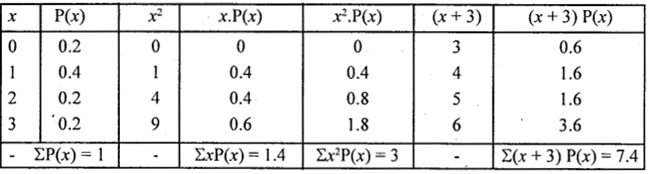∴ E(x) = Σx. P(x) = 1.4
Var (x) = E(x2) – [E(x)]2 = Σx2. P(x) – (1.4)2
= 3 – 1.96= 1.04
E(x + 3) = Σ(x + 3) P(x) = 7.4

Question 41.
If x is a discrete random variable and let a, b be two constants, show that
1. E(a) = a
2. E(ax) = aE(x)
3. E(ax + b) = aE(x) + b
4. Var (a) = 0
5. Var (ax) = a2 Var (x)
6. Var (ax + b) = a2 Var (x).
Proof: From the definitions of probability mass function and mathematical expectation
1. E(a) = Σa .P(a) = a. ΣP(x) = a.l = a. ∵ΣP(x) = 1

2. E(ax) = Σax P(x) = aΣx P(x) = a. E(x) ∵Σx P(x) = E(x)

3. E(ax + b) = E(ax + b). P(x) Removing bracket
= Σax. P(x) + Σb.P(x) = aΣx . P(x) + bΣP(x)
= a. E(x) + b. 1 = aE(x) + b

4. Var (a) = E[a – E(a)]2; ∴ V(x) = E[x – E(x)]2
= E[a – a]2 = E(0) = 0

5. Var (an) = E[ax – E(ax)]2 = E[ax – aE(x)]2; ∴E(ax) = aE(x)
= a2E(x – E(x)]2 = a2 Var (x)

6. Var (ax + b) = E[(ax + b) – E(ax + b)]2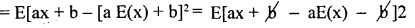= E[ax – aE(x)]2 = a2. E[x – E(x)]2 = a2 Var (x)Question 42.
Define joint/ Bivariate probability function.
Let x and y be two discrete random variables, then P(x, y) is called bivariate or joint probability function of x and y, if P[x = x] and P(y = y)

Question 43.
Define margined probability functionof x.
Ans.
Margined probability function of x: P(x) is obtained from the joint probability faction of P(x,y).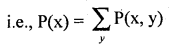Question 44.
Define marginal probability function of y.
Ans.
Marginal probability function of y: P,(y) 1’s obtained from the joint probability function of P(x, y).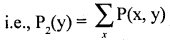Question 45.
State and prove the addition theorem of mathematical expectation. ’
Statement: Let x and y be random variables with respective expectations E(x) and E(y). Then, the expectations of the sum of the random variables is
E(x + y) =E(x) + E(y)
Proof: Let x and y be two discrete random variables, with their joint probability function : P(x, y) as,
P(x,y) =P(x = x, y = y)
The marginal probability distributions of x and y are: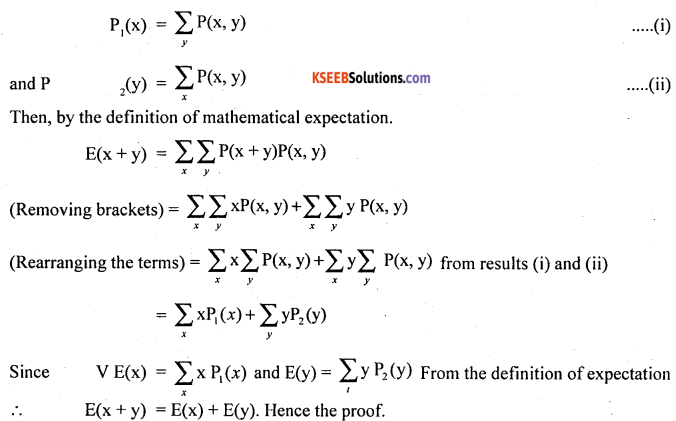Question 46.
State tne prove multiplication theorem of mathematical expectation.
Statement: Let x and y be two independent discrete random variables with respective expectations E(x) and E(y). then the expectation of the product of these random variables is: E(xy) = E(x) . E(y)
Proof: Let x and y be two independent discrete random variables, then joint probability function P(x, y) is
P(x, y) = P1(x). P2(y) ……(i)
Then, by the defintion of mathematical expectation.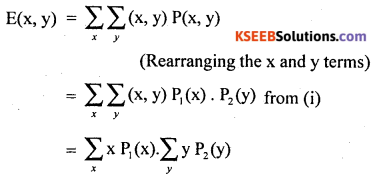From the definition mathematical expectation
E(x y) = E(x) . E(y), Hence the proof.

Question 47.
Find, Mean, Variance of the following probability distribution.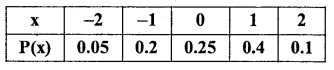Mean = E(x) = Σx . P(x)
= (-2) × 0.05 + (-1) 0.2 + 0 × 0.25 + 1 × 0.4 + 2 × 0.1
= -0.1 – 0.2 + 0 + 0.4 + 0.2 = 0.3
Var(x) =E(x2) – [E(x)]2
E(x2) = Σx2. P(x) = (-2)2 x 0.05 + (-1)2 x 0.2 + 0 x 0.25 + 1 x 0.4 + 2 x 0.1
= 0.2 + 0.2 + 0 + 0.4 + 0.2 = 1
∴ Var (x) = 1 – (0.3)2 = – 0.09 = 0.91

Question 48.
For the following probability distribution find E(x), E(2x + 5), E(x2 + 3), V(x), V(2x).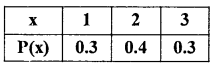E(x) = Σx . P(x)
= 1 × 0.3 + 2 × 0.4 + 3 × 0.3
= 0.3 + 0.08 + 0.9 = 20
and E(x2) = Σx2. P(x)
= 12 × 0.3 + 22 × 0.4 + 32 × 0.3
= 0.3 +1.6+ 2.7 = 4.6
Var (x) = E(x2) – [E(x)]2 = 4.6 – (2)2 = 0.6
E(2x + 5); From the results : E(ax + b) = aE(x) + b
= 2E(x) + 5; Since E(x) = 2
E(2x + 5)=2 × 2 + 5 = 9
E(x2 + 3) = E(x2) + 3 = 4.6 + 3 = 7.6
∴ Var (2x) = 22 Var (x) = 4 x 0.6 = 2.4

Question 49.
From the Following probability distribution. find k, E(x), E$$\left(\frac{x}{3}\right)$$, E(-4x) Var (x) Var (5x) var $$\left(\frac{2 x}{3}\right)^{3}$$, Var (-3x).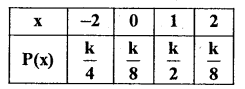We know from the probability mass function. ΣP(x) = 1
$$\therefore \quad \frac{\mathrm{k}}{4}+\frac{\mathrm{k}}{8}+\frac{\mathrm{k}}{2}+\frac{\mathrm{k}}{8}=1$$ $$k\left[\frac{1}{4}+\frac{1}{8}+\frac{1}{2}+\frac{1}{8}\right]=1$$
K.1 = 1; ∴K = 1
The probability distribution reduce now,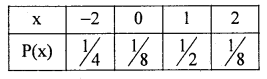$$\mathrm{E}(\mathrm{x})=\Sigma \mathrm{x} . \mathrm{P}(\mathrm{x})=(-2) \times \frac{1}{4}+0 \times \frac{1}{8}+1 \times \frac{1}{2}+2 \times \frac{1}{8}$$
= -0.5 + 0+ 0.5 + 0.25
E(x) = 0.25
$$\mathrm{E}\left(\mathrm{x}^{2}\right)=(-2)^{2} \times \frac{1}{4}+0^{2} \times \frac{1}{8}+1^{2} \times \frac{1}{2}+2^{2} \times \frac{1}{8}$$
= 1 + 0 + 0.5 + 0.5 = 2
Var (x) = E(x2) – [E(x)]2
= 2 – (0.25)2 = 2 – 0.0625 = 1.9375
$$\mathrm{E}\left(\frac{\mathrm{x}}{3}\right)=\mathrm{E}\left(\frac{1}{3} \cdot \mathrm{x}\right)$$; From E(ax) = aE(x)
$$=\frac{1}{3} \mathrm{E}(\mathrm{x})=\frac{1}{3} \times 0.25=0.083$$
E(-4x) = -4E()x = -4 × 0.25 = -1
Var (5x) = 52 var (x); from Var (ax) = a2 Var (x)
= 25 × 1.9375 = 48.4375
Var$$\left(\frac{2 x}{3}\right)$$ = Var$$\left(\frac{2}{3} \cdot x\right)$$
= $$\left(\frac{2}{3}\right)^{2}$$Var(x) = $$\frac { 4 }{ 9 }$$ x 1.9375 = 0.861
Var (-3x) = (-3)2 Var (x) = 9 × 1.9375 = 17.4375Question 50.
A purse has 5 Ten rupee coins, 3 five rupee coins and 2 two rupee coins. A coin is drawn at random from the purse. Find expectation of amount draw.
Let x denote the expected amount drawn, is a discrete random variable which takes the values : x = 10, 5, 2 with the prababilities
P(x = 10) = P(drawing ₹ 10 coin) = $$\frac { 5 }{ 10 }$$
P(x=5)= $$\frac { 3 }{ 10 }$$. P(x = 2)=$$\frac { 2 }{ 10 }$$
Then, the probability distribution is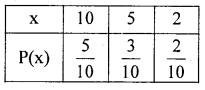Expected amount draw : E(x) = Ex. P(x)
$$=10 \times \frac{5}{10}+5 \times \frac{3}{10}+2 \times \frac{2}{10}=\frac{50}{10}+\frac{15}{10}+\frac{4}{10}$$ = ₹ 6.90

Question 51.
In a lottery there are 1000 tickets costing ₹5 each. There is one first prize worth ₹ 1000. Two second prizes worth ₹ 500 each and ten third prizes worth ₹ 100 each. Find expected gain in buying one lottery ticket.
Let x denote the expected gain is a discrete random variable which takes the values x : 995, 495, 95 and -5. Since he buys a ticket of for ₹ 5 is deducted). The respective probability can be calculated as below:
p(x = 995) = $$\frac { 1 }{ 1000 }$$: p(x = 495) = $$\frac { 2 }{ 1000 }$$
p(x = 95) = $$\frac { 10 }{ 1000 }$$ p(x = -5) = $$\frac { 987 }{ 1000 }$$
The probability distribution is :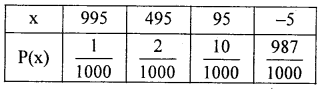Expected gain : E(x) = Σx. P(x)
$$=995 \times \frac{1}{1000}+495 \times \frac{2}{1000}+95 \times \frac{10}{1000}-5 \times \frac{987}{1000}$$
= 0.995 + 0.99 + 0.95 – 4.985 = -2.47
i.e., expected loss in buying a lottery ticket is ₹ 247.

Question 52.
A person enters into a fair by paying ₹10. In the fair if choose to hit a target with airgun by paying ₹ 1 per shot if he hits gets ₹ 50. The probability of hitting a target is 1/2. Find the expect amount.
Let x denote the expect amount is a discrete random variable, which takes the values : -11, 39.
The respective probabilities
P(x = -11) = P(not hitting a target) = $$\frac { 2 }{ 3 }$$
P(x = 39) = P(hitting a target) = $$\frac { 1 }{ 3 }$$
Therefore, the expected amount is :
E(x) = Σx. P(x)
= -11 × $$\frac { 2 }{ 3 }$$ + 39 × $$\frac { 1 }{ 3 }$$ = -7.33 + 13 = Rs. 5.67 profit.

Question 53.
Find expected sum of numbers obtained in a throw of two dice.
Let x and y be the numbers appeared on the faces of two dice is a discrete random variable, then each die show with numbers 1, 2,3, 4, 5, 6 with respective probabilities $$\frac { 1 }{ 6 }$$ each.
Expected number obtained on the first die be : E( x) = Σx . P(x)
$$=1 \times \frac{1}{6}+2 \times \frac{1}{6}+3 \times \frac{1}{6}+\ldots \ldots+6 \times \frac{1}{6}=3.5$$
Similarly, expected number on the faces of 2nd die be E(y) = 3.5
The expectation of sum of numbers obtained is
E(x + y) = E(x) + E(y) = 3.5 + 3.5 = 7
Note: Similarly, sum of’ ‘n’ dice of numbers can be calcualted.

Question 54.
A bag has ten tiny of dice. Two dice are randomly drawn. Find the expectation of the product of the numbers obtained on the faces of dice.
Let x and y denoted the numbers obtained on the faces of dice, is a discrete random variable with values 1, 2,…. 6 and with the probability $$\frac { 1 }{ 6 }$$ each.
Therefore E(x) = $$1 \times \frac{1}{6}+2 \times \frac{1}{6}+\ldots \ldots+6 \times \frac{1}{6}=3.5$$
Similarly, E(y) =3.5
Since, drawing dice are independens, therefore
E(x, y) = E(x) . E(y) = 3.5 × 3.5 = 12.25
Note: Similarly product of’n’ dice of numbers can also be calculated in the square way.

Question 55.
If in a bivoriate data, E(x) = 4, E(x2) = 25, E(y) = 8 and E(y2) = 100 and E(x y ) = 24. Find Var (x), Var (y), Cov (x, y) and the coefficient of correlation (3xy).
Var (x) = E(x2) – [E(x)]2 = 25 – 42 = 9
Var (x) = E(y2) – [E(y)]2 = 100 – 82 = 36
COV (x, y) = E(xy) – E(x) . E(y) = 24 – 4 x 8 = -8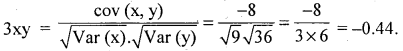Question 56.
In a bivariate data E(x) = 1.3. E(x2) = 3.4, E(y) = 0 and E(y2) = 4. E(xy) = 0.1 Find S.D(x), SD(y) and rxy.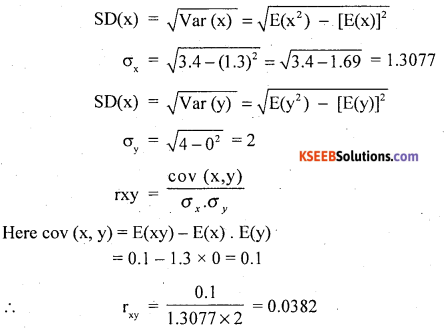Question 57.
For the following bivariate probaiblity distribution, find E(x + y) and E(xy).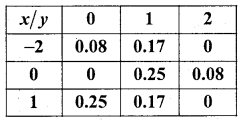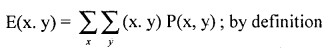Here 0.08, 0.17, 0.25, 0.08, 0.25 and 0.17 are joint probability function P(x, y) of discrete random variables x and y.
E(x.y) =(-2) × 0 × 0.08 + 0 × 0 × 0+1 × 0 × 0.25 + (-2) × 1 × 0.17 + 0 × 1 × 0.25 + 1 × 1 × 0.17 +(2) × 2 × 0 + 0 × 2 × 0.08+ 1 × 2 × 0.
= (0 + 0 + 0) +(0.34 + 0 + 0.17) + (0 + 0 + 0)
∴ E(x.y) =0.17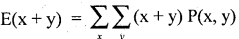= (-2 + 0) 0.08 + (0 + 0) 0 + (1 + 0) 0.25 + (-2 + 1) 0.17 + (0 + 1)0.25 + (1 + 1)0.17+ (-2 +2) +0 + (0 + 2) 0.08+ (1 +2)0 = (-0.16 + 0 + 0.25) + (-0.17 + 0.25 + 0.34) + (0 + 0.16 + 0)
= 0.09 + 0.42 + 0.16.
E(x + y) =0.67Question 58.
From the following bivariate probability distribution, find the coefficient of correlation.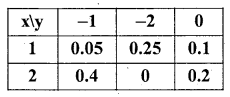The coefficient of correlation is :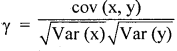Here Var(x) = E(x2) – [E(x)]2
‘To calculate, obtain the marginal probility distribution of x: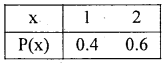Here : 0.05 + 0.25 + 0.1 = 0.4 and 2 : 0.4 + 0 + 0.2 = 0.6
E(x) = Ex . P(x) = 1 × 0.4 + 2 × 0.6 = 1.6
E(x2) = Ex2. P(x) = 12 × 0.4 + 22 × 0.06 = 2.8
∴ Var (x)= 2.8 — (1.6)2 = 0.24

The marginal probability distribution of y: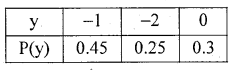E(y) = Ey P(y) = (-1) × 0.45 + (-2) × 0.25 + 0 × 0.3 = – 0.95
E(y2) = (-1 )2 × 0.45 + (-2)2 × 0.25 + 02 × 0.3 = 0.45 + 1 + 0 = 1.45
∴ Var(y) = E(y2) – [E(y)]2 = 1.45 – (-0.95)2 = 1.45 – 0.9025 = 0.5475
cov (x, y) = E(xy) – E(x) . E(y)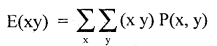= (1 × (-1) × 0.05) + (2 × (-1) × 0.4) + (1 × (-2) × 0.25) + (2 × (-2) × 0) + (1 × 0 × 0.1) +(2 × 0 + 0.2)
= -0.05 -0.8 -0.5 + 0 + 0 + 0
E(xy) =-1.35
∴ cov(x,y) =-1.35- 1.6 x (-0.95) = -1.35 + 1.52 = -0.17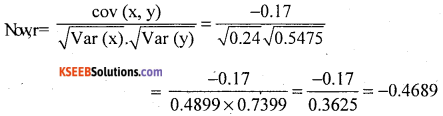Question 59.
If E(x2) = 65, and E(x) = 4. Find standard deviation of x.
Here,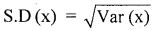Var(x) = E(x2) = [E(x)]2 = 65 – (42) = 65 – 16 = 49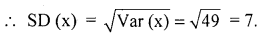Question 60.
IfVar(x)=0.4327, and E(x2) = 0.75. Find E(x).
Var(x) = E(x2) – [E(x)]2
0.4327 = 0.75 – [E(x)]2
[E(x)]2 =0.75-0.4327 = 0.3153
∴ E(x) = $$\sqrt{0.3153}$$ =0.5615

Question 61.
If x and y be two independent random variable with E(xy) = 8, and E(x) = 2, then find E(y).
Since x and y are independent then E(xy) = E(x). E(y)
i.e., 8 = 2. E(y) ∴ E(y) = 2

Question 62.
For a bivariate data if E(x) = 5, E(y) = 3, E(x2) = 45, E(y2) = 85 and E(x) = 18. Find yxy.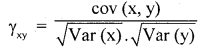so, Var (x) = E(x2) = [E(x)]2 = 45 – (5)2 = 45 – 25 = 20
Var (y) = E(y2) – [(y)]2 = 85 – (3)2 = 85 – 9 = 76
COV (x, y) = E(xy) – E(x) . E(y) =18-5 x 3 = 18- 15 = 3
∴ $$\gamma=\frac{3}{\sqrt{20} \times \sqrt{76}}=\frac{3}{4.472 \times 8.718}=\frac{3}{38.987}=0.0769$$

Question 63.
If E(x) = 5 and E(y) = 12, find E(x + y), E(x – y), E(4x + 2y), and E(-3x + 2y).
E(x + y) = E(x) + E(y) , by theorem = 5 + 12= 17
E(x-y) = E(x) – E(y) = 5 – 12 = -7
E(4x + 2y) = 4E(x) + 2E(y) = 4 × 5 + 2 × 12 = 20 + 24 = 44
E(-3x + 2y) = (-3) E(x) + 2E(y) = (-3) × 5 + 2 × 12 = 15 + 24 = 9

Question 64.
If Var (x) = 3, find Var (5x), Var (-8x), Var (-x), Var (4x – 5) and Var (8 – 3x)